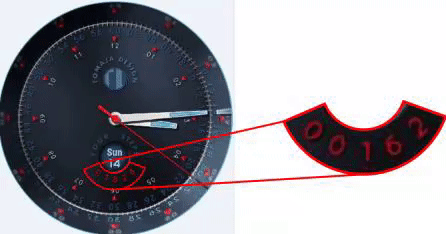# [TOMAJA Mini Tutorial] S T E P S Digits - Isolated Ones, Tens, Hundreds, Thousands & Ten Thousands

Hello there,

I wanted to have an active arch down transformed steps field with text circle. I could not find any calculation according to separated step digits, so I prepared one ;). Here is the result:And how you could get there:

Calculation of Ones:

(#ZSC#-(floor(#ZSC#/10000)) * 10000-((floor(#ZSC#/1000))-((floor(#ZSC#/10000)) * 10)) * 1000-(floor(((#ZSC#-((floor(#ZSC#/10000)) * 10000))-(((floor(#ZSC#/1000))-((floor(#ZSC#/10000)) * 10)) * 1000))/100)) * 100-(floor((#ZSC#-(floor(#ZSC#/10000)) * 10000-((floor(#ZSC#/1000))-((floor(#ZSC#/10000)) * 10)) * 1000-(floor(((#ZSC#-((floor(#ZSC#/10000)) * 10000))-(((floor(#ZSC#/1000))-((floor(#ZSC#/10000)) * 10)) * 1000))/100)) * 100)/10)) * 10)

Calculation of Tens:

(floor((#ZSC#-(floor(#ZSC#/10000)) * 10000-((floor(#ZSC#/1000))-((floor(#ZSC#/10000)) * 10)) * 1000-(floor(((#ZSC#-((floor(#ZSC#/10000)) * 10000))-(((floor(#ZSC#/1000))-((floor(#ZSC#/10000)) * 10)) * 1000))/100)) * 100)/10))

Calculation of Hundreds

(floor(((#ZSC#-((floor(#ZSC#/10000)) * 10000))-(((floor(#ZSC#/1000))-((floor(#ZSC#/10000)) * 10)) * 1000))/100))

Calculation for Thousands

((floor(#ZSC#/1000))-((floor(#ZSC#/10000)) * 10))

Calculation for Ten Thousands

(floor(#ZSC#/10000))

I did not published this particular fine-tuned watch face design yet (Jan, 14, 2018) however you could probably find it in my collection soon.

WARNING: There is a limit of 99,999 Steps in those expressions. So, if you walk more than 99,999 steps a day, you will get incorrect result.

1 Like

Well, now I find out, that there is also an another and much elegant way to separate digits at the step #ZSC# tag from @eradicator09.

So, if you are interesting in, forget about this mini tutorial and use this link please.

1 Like

Hmmmm, just curious … is it possible to make 1/10 Steps? I know it makes no sense but i am working on a pedometer…

What do you mean? Can you give an example?

Well i am creating a classic car display at he moment and down below there is a pedometer. I want the Number in the white field in 1/10 steps. At the moment, there is a placeholder with second.

Example:

Greeeeeetings, GAUSS.

1 Like

…so you would like to have 0.1 steps, correct? hmmm… it is hard to know in the front when the next step will comes…

Basically we could work with a kind of average value (general fix value, day average, minute average, …)

At the moment only a “fancy average” could be realized… however I do not know how to display correct steps value at the same time.

Next point would be that the steps (usually) do not come in the real time, but only after few seconds…

If I would like to have such an instrument implemented into my watch face, I would go the "interpAccel way"as I use it now for changing fitness indicator